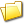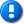home | career | drupal | java | mac | mysql | perl | scala | uml | unix

# Java example source code file (AbstractCurveFitter.java)

This example Java source code file (AbstractCurveFitter.java) is included in the alvinalexander.com "Java Source Code Warehouse" project. The intent of this project is to help you "Learn Java by Example" TM.

## Java - Java tags/keywords

abstractcurvefitter, collection, leastsquaresoptimizer, leastsquaresproblem, levenbergmarquardtoptimizer, multivariatematrixfunction, multivariatevectorfunction, parametricunivariatefunction, theoreticalvaluesfunction, util, weightedobservedpoint

## The AbstractCurveFitter.java Java example source code

```/*
* Licensed to the Apache Software Foundation (ASF) under one or more
* contributor license agreements.  See the NOTICE file distributed with
* The ASF licenses this file to You under the Apache License, Version 2.0
* (the "License"); you may not use this file except in compliance with
* the License.  You may obtain a copy of the License at
*
*
* Unless required by applicable law or agreed to in writing, software
* WITHOUT WARRANTIES OR CONDITIONS OF ANY KIND, either express or implied.
* See the License for the specific language governing permissions and
*/
package org.apache.commons.math3.fitting;

import java.util.Collection;

import org.apache.commons.math3.analysis.MultivariateVectorFunction;
import org.apache.commons.math3.analysis.MultivariateMatrixFunction;
import org.apache.commons.math3.analysis.ParametricUnivariateFunction;
import org.apache.commons.math3.fitting.leastsquares.LeastSquaresOptimizer;
import org.apache.commons.math3.fitting.leastsquares.LeastSquaresProblem;
import org.apache.commons.math3.fitting.leastsquares.LevenbergMarquardtOptimizer;

/**
* Base class that contains common code for fitting parametric univariate
* real functions <code>y = f(pi;x), where {@code x} is
* the independent variable and the <code>pi are the
* <em>parameters.
* <br/>
* A fitter will find the optimal values of the parameters by
* <em>fitting the curve so it remains very close to a set of
* {@code N} observed points <code>(xk, yk),
* {@code 0 <= k < N}.
* <br/>
* An algorithm usually performs the fit by finding the parameter
* values that minimizes the objective function
* <pre>```
*  ∑y<sub>k - f(xk)2,
* </code>``````
``` * which is actually a least-squares problem. * This class contains boilerplate code for calling the * {@link #fit(Collection)} method for obtaining the parameters. * The problem setup, such as the choice of optimization algorithm * for fitting a specific function is delegated to subclasses. * * @since 3.3 */ public abstract class AbstractCurveFitter { /** * Fits a curve. * This method computes the coefficients of the curve that best * fit the sample of observed points. * * @param points Observations. * @return the fitted parameters. */ public double[] fit(Collection<WeightedObservedPoint> points) { // Perform the fit. return getOptimizer().optimize(getProblem(points)).getPoint().toArray(); } /** * Creates an optimizer set up to fit the appropriate curve. * <p> * The default implementation uses a {@link LevenbergMarquardtOptimizer * Levenberg-Marquardt} optimizer. * </p> * @return the optimizer to use for fitting the curve to the * given {@code points}. */ protected LeastSquaresOptimizer getOptimizer() { return new LevenbergMarquardtOptimizer(); } /** * Creates a least squares problem corresponding to the appropriate curve. * * @param points Sample points. * @return the least squares problem to use for fitting the curve to the * given {@code points}. */ protected abstract LeastSquaresProblem getProblem(Collection<WeightedObservedPoint> points); /** * Vector function for computing function theoretical values. */ protected static class TheoreticalValuesFunction { /** Function to fit. */ private final ParametricUnivariateFunction f; /** Observations. */ private final double[] points; /** * @param f function to fit. * @param observations Observations. */ public TheoreticalValuesFunction(final ParametricUnivariateFunction f, final Collection<WeightedObservedPoint> observations) { this.f = f; final int len = observations.size(); this.points = new double[len]; int i = 0; for (WeightedObservedPoint obs : observations) { this.points[i++] = obs.getX(); } } /** * @return the model function values. */ public MultivariateVectorFunction getModelFunction() { return new MultivariateVectorFunction() { /** {@inheritDoc} */ public double[] value(double[] p) { final int len = points.length; final double[] values = new double[len]; for (int i = 0; i < len; i++) { values[i] = f.value(points[i], p); } return values; } }; } /** * @return the model function Jacobian. */ public MultivariateMatrixFunction getModelFunctionJacobian() { return new MultivariateMatrixFunction() { /** {@inheritDoc} */ public double[][] value(double[] p) { final int len = points.length; final double[][] jacobian = new double[len][]; for (int i = 0; i < len; i++) { jacobian[i] = f.gradient(points[i], p); } return jacobian; } }; } } } Other Java examples (source code examples) Here is a short list of links related to this Java AbstractCurveFitter.java source code file:The search pageOther Java source code examples at this package levelClick here to learn more about this project ```